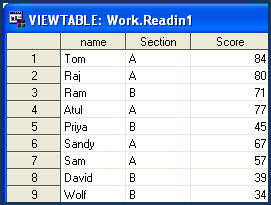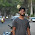# SAS : Where Statement and Dataset Options

The WHERE statement is an alternative to IF statement when it comes to subsetting a data set.

Basic Data Subsetting

Syntax of WHERE statement :

WHERE (condition is true) => It means subsetting a dataset.

Comparison Operators

 Symbolic Mnemonic Meaning Example = EQ equals WHERE gender = ‘M’; or WHERE gender EQ ‘M’; ^= or ~= NE not equal WHERE salary NE . ; > GT greater than WHERE salary GT 4500; < LT less than WHERE salary LT 4500; >= GE greater than or equal WHERE salary GE 4500; <= LE less than or equal WHERE salary LE 4500; in IN selecting multiple values WHERE country IN(‘US’ ’IN’);

Task1 : Suppose you want to select only section A students. You know the variable Section contains information for students' sections.

where section EQ "A" => This would tell SAS to select only section A values.

You can also write where section = "A". This statement serves the same purpose.

The output is shown below :

LOGICAL OPERATORS

 Symbolic Mnemonic Meaning Example & AND Both conditions true WHERE gender =’M’ and age =1; | OR Either condition true WHERE gender =’M’ or age =1; ~ or ^ NOT Reverse the statement WHERE country not IN(‘US’,’IN’);

Task2 : Suppose you want to select section A and B students. You know the variable Section contains information for students' sections.

where section IN ("A" "B") => This would tell SAS to select section A and B values.

However, you can also write ...

The output is shown below :BETWEEN-AND Operator : Between Two Numbers

Task 3 : Suppose you want to select scores whose values are greater than or equal to 50 and less than or equal to 75.

where Score between 50 and 75 => This would tell SAS to select values through 50 and 75 (not 51 to 74).

This can also be written like :

where Score GE 50 and Score LE 75;

IS MISSING Operator : Selecting Missing Values

Task 4 : Suppose you want to select only those observations in which students did not fill their section information.

The dataset is modified to include missing values in SECTION variable.

Where section is missing => This would tell SAS to select missing values for variable SECTION.

The output is shown below :

IS NOT MISSING Operator : Selecting Non-Missing Values

Task 5 : Suppose you want to select only those observations in which students filled their section information.

Where section is not missing => This would tell SAS to select non-missing values.

The output is shown below :

The NOT operator can be used within WHERE statement in many ways :

1. where section is missing and score is not missing;

2. where not (score in (34,44,84));

3. where not (Score between 50 and 75);

4. where NOT(Section EQ "A");

CONTAINS Operator : Searching specific character

Task 6 : Suppose you want to select only those observations in which students' name contain 'hul'.

where name contains 'hul' => This would tell SAS to select observations having the values Rahul, Sahul and Lahul for the variable NAME.

Note : The CONTAINS operator is case sensitive.

Since the CONTAINS operator is case sensitive, where Name contains 'HUL' would not select any observation. The log for this statement is shown below :LIKE Operator : Pattern Matching

The LIKE operator selects observations by comparing the values of a character variable to a specified pattern. It is case sensitive.

Task7 : To select all students with a name that starts with the letter S.

There are two special characters available for specifying a pattern:

1. percent sign (%) - Wildcard Character

2. underscore ( _ ) - Fill in the blanks

where name like 'S%';

OR

where name like 'Sa%';

In this dataset, the above statements would produce the same result :

Examples :

1. where name like '_am';

You can also write this statement like .....

where name like '%am';

2.  where name like '_ahu_';

This would not select PAHULK from the variable NAME.

3. where name like '_ahu__';

This would select PAHULK as double underscore (__) is stated.

Sounds-like Operator : Selecting sound like characters

Task8 : To select names that sound like 'Ram'.

The output is shown below :

WHERE = Data Set Option

1. In the example shown below, the WHERE= data set option is used to select only section A data.

data readin1 (where = (section ='A'));
run;

2. The following example shows how to use WHERE= data set option in procedures

run;

In this case, you can also use WHERE statement....

where section='A';
run;

Difference between WHERE and IF conditions - WHERE Vs. IF .Share
Related PostsDeepanshu founded ListenData with a simple objective - Make analytics easy to understand and follow. He has over 10 years of experience in data science. During his tenure, he has worked with global clients in various domains like Banking, Insurance, Private Equity, Telecom and Human Resource.

9 Responses to "SAS : Where Statement and Dataset Options"
1.Hi Deepanshu!
I have collected data on change in educational practices using a questionaire and wish to do the analysis. What all types of analysis can be done using SAS?

2.3.Hello ! What is the difference between below 2 snippets of code?
/*code 1*/
data readin1 (where = (section ='A'));
run;

/*code 2*/
set readin (where = (section ='A'));
run;

I think code2 is more efficient while dealing with large datasets as it subsets the dataset at reading stage only.

1.yes your understanding is correct, instead of reading whole dataset and then filtered out , run your logic in options like code 2. It will only read where condition is satisfied.

4.Very informative

5.Hey in one of your examples of where name = "_ahu__" would Rahul be selected because after l there is no other character available.

6.Can you send me sas back end process how data reads in sas

7.Hi may i know how to use between operator for character data.

8.I think we can't use between operator for character data because we can't range it from this to this..

Next → ← Prev